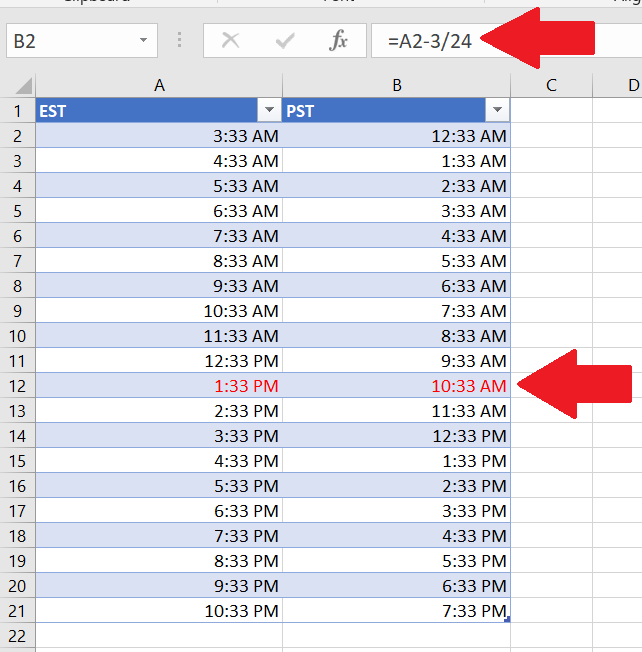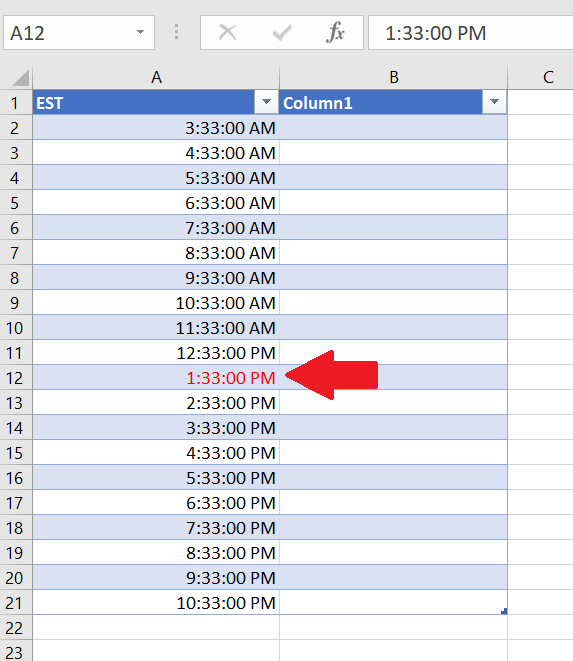# How to convert EST to PST

In this tutorial we’ll learn about time zone conversion of EST(Eastern Standard Time) to PST (Pacific Standard Time). Before doing it, one should know the difference between time zones then you would be able to build your own time zone converter. Eastern Standard Time is 3 hours  ahead of Pacific Standard Time.Microsoft Excel is a software that can also help us in mathematical calculations along with data analysis. There are amazing features of Microsoft Excel which helps a lot in day to day routine. For example, it can help us in time zone conversion too with a very simple method.

### Step 1 – Select the Cell Format– Open Excel and select the blank sheet.
– Choose a suitable cell where you wish to type the UTC time. In this case we have chosen A2.
– Press the shortcut key Ctrl + 1 to set the cell format to TIME from General.
– A dialogue box will appear, select the cell type as TIME, then select the type given at the right side.
– Click OK as shown in picture above;

### Step 2 – Type the EST– Type in any time value that you wish to convert from UTC to PST in A2.
– Drag it down a few rows to create some data samples.
– In this instance, we started the EST time from 3:33:00 A.M. to 10:3:00 P.M.
– Excel will automatically increment in hours.
– The desired result is shown in the picture above;

### Step 3 – Implement the formula– Choose a suitable cell where you wish to implement the formula. In this case we have chosen B2 to implement this formula.
– As we know PST is 3 hours behind EST therefore, we will subtract 3 hours from EST time.
– However, we will have to divide with 24 to handle the A.M to P.M conversion properly otherwise incorrect results will be produced.
– Therefore, =A2 – 3 / 24 will be the formula, to be used in the cell B2.
– Implement the formula and hit enter and you will see the PST converted time in cell B2.
– Now drag down with the help of the handle till the last entry of time range in column A to implement the formula to all the entries.
– All time values of EST in column A are now converted to PST and displayed in column B, just like the picture shown above.

So this is how you can create a Time Zone Calculator in Microsoft Excel in a very simple way.